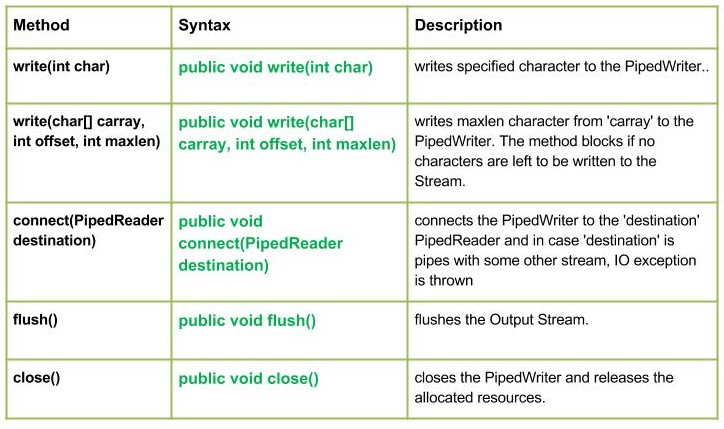# Java.io.PipedWriter Class in Java

• Last Updated : 23 Jan, 2017This class is basically a piped character-output streams. In I/O Piped, simply means a link between two threads running in JVM at the same time. So, Pipes are used both as source or destination.
A pipe is said to be broken if a thread that was providing data bytes to the connected piped output stream is no longer alive.

Declaration:

```public class PipedWriter
extends Writer```

Constructor :

• PipedWriter() : creates a PipedWriter, that it is not connected.
• PipedWriter(PipedReader inStream) : creates a PipedWriter, that it is connected to PipedReader – ‘inStream’.

Methods:

• write(int char) : java.io.PipedWriter.write(int char) writes specified character to the PipedWriter.
Syntax :

```public void write(int char)
Parameters :
char : character to be written
Return :
void
Exception :
-> IOException : if in case IO error occurs.```
• write(char[] carray, int offset, int maxlen) : java.io.PipedWriter.write(char[] carray, int offset, int maxlen) writes maxlen character from ‘carray’ to the PipedWriter. The method blocks if no characters are left to be written to the Stream.
Syntax :

```public void write(char[] carray, int offset, int maxlen)
Parameters :
carray : data of the carray
offset : starting in the destination array - 'carray'.
maxlen : maximum length of array to be read
Return :
void
Exception :
-> IOException : if in case IO error occurs.```

Implementation :

 `// Java program illustrating the working of PipedWriter``// write(char[] carray, int offset, int maxlen)`` ` `import` `java.io.*;``public` `class` `NewClass``{``    ``public` `static` `void` `main(String[] args) ``throws` `IOException``    ``{``        ``PipedReader geek_reader = ``new` `PipedReader();``        ``PipedWriter geek_writer = ``new` `PipedWriter();`` ` `        ``// Use of connect() : connecting geek_reader with geek_writer``        ``geek_reader.connect(geek_writer);`` ` `        ``char``[] carray = {``'J'``, ``'A'``, ``'V'``, ``'A'``};`` ` `        ``// Use of write(char[] carray, int offset, int maxlen)``        ``geek_writer.write(carray, ``0``, ``4``);``        ``int` `a = ``5``;``        ``System.out.print(``"Use of write(carray, offset, maxlen) : "``);``        ``while``(a>``0``)``        ``{``            ``System.out.print(``" "` `+ (``char``) geek_reader.read());          ``        ``}``       ` `    ``}``}`

Output :

`Use of write(carray, offset, maxlen) :  J A V A`
• close() : java.io.PipedWriter.close() closes the PipedWriter and releases the allocated resources.
Syntax :

```public void close()
Parameters :
--------------
Return :
void
Exception :
-> IOException : if in case IO error occurs.```
• connect(PipedReader destination) : java.io.PipedWriter.connect(PipedReader destination) connects the PipedWriter to the ‘destination’ PipedReader and in case ‘destination’ is pipes with some other stream, IO exception is thrown
Syntax :

```public void connect(PipedReader destination)
Parameters :
destination : the PipedReader to be connected to
Return :
void
Exception :
-> IOException : if in case IO error occurs.```
• flush() : java.io.PipedWriter.flush() flushes the Output Stream.
Syntax :

```public void flush()
Parameters :
------------
Return :
void
Exception :
-> IOException : if in case IO error occurs.```

Java code illustrating the working of PipedWriter class methods :

 `// Java program illustrating the working of PipedWriter``// write(), connect``// close(), flush()`` ` `import` `java.io.*;``public` `class` `NewClass``{``    ``public` `static` `void` `main(String[] args) ``throws` `IOException``    ``{``        ``PipedReader geek_reader = ``new` `PipedReader();``        ``PipedWriter geek_writer = ``new` `PipedWriter();``        ``try``        ``{``            ``// Use of connect() : connecting geek_reader with geek_writer``            ``geek_reader.connect(geek_writer);`` ` `            ``// Use of write(int byte) :``            ``geek_writer.write(``71``);``            ``geek_writer.write(``69``);``            ``geek_writer.write(``69``);``            ``geek_writer.write(``75``);``            ``geek_writer.write(``83``);`` ` `            ``// Use of flush() method :``            ``geek_writer.flush();``            ``System.out.println(``"Use of flush() method : "``);`` ` `            ``int` `i = ``5``;``            ``while``(i > ``0``)``            ``{``                ``System.out.print(``" "` `+ (``char``) geek_reader.read());``                ``i--;``            ``}`` ` `            ``// USe of close() method :``            ``System.out.println(``"\nClosing the Writer stream"``);``            ``geek_writer.close();`` ` `        ``}``        ``catch` `(IOException excpt)``        ``{``            ``excpt.printStackTrace();``        ``}``    ``}``}`

Output :

```Use of flush() method :
G E E K S
Closing the Writer stream```

Next Article: Java.io.PipedReader Class in Java

This article is contributed by Mohit Gupta 🙂. If you like GeeksforGeeks and would like to contribute, you can also write an article using contribute.geeksforgeeks.org or mail your article to contribute@geeksforgeeks.org. See your article appearing on the GeeksforGeeks main page and help other Geeks.
Please write comments if you find anything incorrect, or you want to share more information about the topic discussed above.

My Personal Notes arrow_drop_up